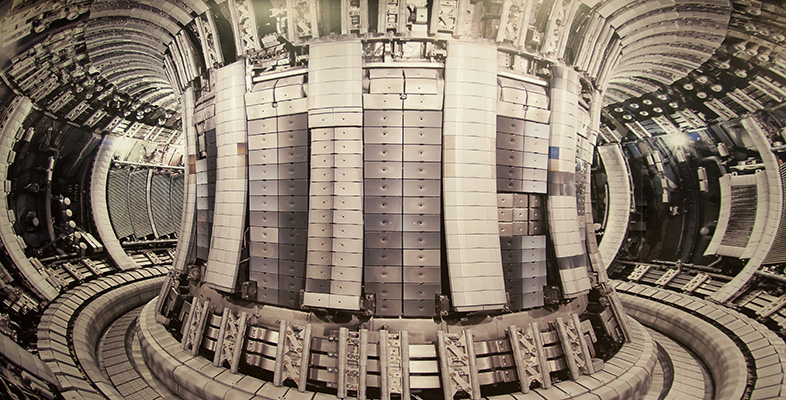Unclear about nuclear?

Start this free course now. Just create an account and sign in. Enrol and complete the course for a free statement of participation or digital badge if available.

Free course

# 2.1.1 Electrons, protons, neutrons and isotopes

Whatever the type of atom, each one has certain features in common. Each contains a central nucleus (plural nuclei), which carries a positive electric charge as well as most of the atom’s mass. The nucleus is surrounded by one or more negatively charged particles known as electrons (symbol: e), each of which has a much lower mass than the nucleus. The very simplest atoms of all, those of the element hydrogen, have a nucleus consisting of just a single particle, known as a proton (symbol: p). The next simplest atom, helium, has two protons in its nucleus; lithium has three protons; beryllium has four; boron, five; carbon, six; and so on.

The number of protons in the nucleus of an atom is known as its atomic number (Z) and it is this number that determines the chemical element. Elements are defined by their chemistry, and chemistry is all about the interactions of the electrons of the atom, not the nuclei. The number of electrons in a neutral atom is always the same as the number of protons in its nucleus.

When speaking of the value of the electric charge of particles and atoms, it is most convenient to use the charge of a proton as the reference point. So, the electric charge of a proton is said to be ‘+1’ unit. The electric charge of an electron is exactly the same as that of a proton, but negative instead of positive. The electric charge of an electron is therefore ‘−1’ unit.

• What is the atomic number of carbon? What is the electric charge of a carbon nucleus?

• The nucleus of a carbon atom contains six protons, so the atomic number of carbon is 6 and the charge of the nucleus is +6 units.

The other constituents of atomic nuclei are particles known as neutrons (symbol: n) as they have zero electric charge. Neutrons have a mass that is almost, but not exactly, the same as that of protons, which is approximately two thousand times the mass of the electron. They therefore contribute to the mass of an atom, but not to its electric charge.

Normal hydrogen atoms do not have neutrons in their nuclei, although there is a form of hydrogen – known as deuterium – that does. The nucleus of a deuterium atom consists of a proton and a neutron. It is still the element hydrogen (because it contains only one proton) but it is a ‘heavy’ form of hydrogen, thanks to the extra neutron. Deuterium is said to be an isotope of hydrogen. Similarly, normal helium atoms contain two neutrons in their nucleus, along with the two protons; but a ‘light’ isotope of helium contains only one neutron instead. The total number of protons and neutrons in the nucleus of an atom is known as the mass number (A) of the atom. Different isotopes of an element have different mass numbers but their atomic numbers, are the same.

## Question 3

Calculate the mass numbers of:

• a.normal hydrogen
• b.‘heavy’ hydrogen (i.e. deuterium)
• c.normal helium
• d.‘light’ helium.

### Answer

• a.The nucleus of normal hydrogen contains one proton, so the mass number is 1.
• b.The nucleus of deuterium contains one proton and one neutron, so the mass number is 2.
• c.The nucleus of normal helium contains two protons and two neutrons, so the mass number is 4.
• d.The nucleus of ‘light’ helium contains two protons and one neutron, so the mass number is 3.

As a shorthand, isotopes of each element may be represented by using the following notation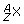, where X is the symbol for the element itself and two numbers are used to indicate the atomic number (lower number, Z) and mass number (upper number, A). So a normal hydrogen atom is represented as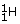and an atom of the heavier isotope, deuterium, as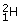. Isotopes of some other light atoms are shown in Table 3 and Figure 7. An alternative notation is to use the name of the element followed by a hyphen and then the mass number. For example, helium would usually be denoted by helium-4, but the lighter isotope referred to above would be given as helium-3. These can also be abbreviated to just the chemical symbol and mass number, for example He-4 and He-3.

Sometimes, protons and neutrons are collectively referred to as nucleons because both types of particle reside inside the nucleus of an atom. Similarly, electrons, protons and neutrons are often collectively referred to as subatomic particles, for obvious reasons. This nomenclature is summarised in Table 4.

## Table 3 Some isotopes of the eight lightest elements. Isotopes of the same element have the same atomic number but a different mass number.

 Atomic number Mass number Isotope name* Isotope symbol 1 1 hydrogen2 deuterium2 3 helium-3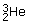4 helium-4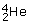3 7 lithium-7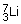4 7 beryllium-7 (unstable)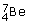8 beryllium-8 (unstable)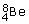9 beryllium-9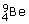5 11 boron-11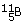6 12 carbon-12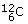13 carbon-13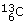14 carbon-14 (unstable)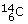7 14 nitrogen-14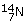8 16 oxygen-16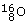* Some isotopes are unstable, that is they decay to other products. The stability of isotopes is discussed in Section 2.2.

Although not needed for a discussion of nuclear energy, it is worth mentioning that protons and neutrons do themselves have an internal structure and are comprised of even smaller particles, known as quarks.

Atoms tend towards a state of being electrically neutral. That is to say, the positive electric charge of the nucleus is exactly balanced by the negative electric charge of the electrons surrounding it. Because each electron carries an electric charge of −1 unit and each proton carries an electric charge of +1 unit, the number of electrons in a neutral atom is exactly the same as the number of protons in its nucleus. However, under some circumstances, an electron might be removed from a neutral atom, or an atom might acquire an ‘extra’ electron, leaving the atom either positively or negatively charged. In this case the atom is known as an ion and the process is that of ionisation. You will learn about ionising radiation later.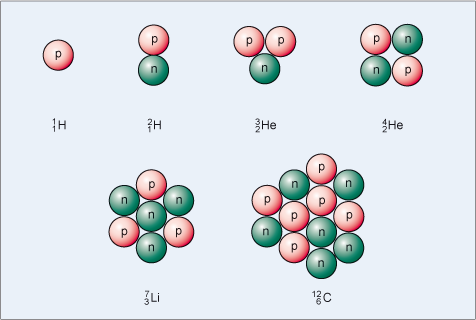Figure 7 Schematic diagrams of the nuclei of some isotopes. Protons are coloured red and labelled with p, and neutrons green and labelled with n.

## Table 4 The constituents of atoms: subatomic particles.

 Electric charge Notes Electron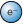−1 unit In a neutral atom, number of electrons = number of protons Nucleons: Mass number = number of nucleons Proton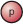+1 unit Atomic number = total number of protons Neutron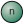0 Isotopes of the same elements have different numbers of neutrons
• What is the difference between an atom of lithium-7 and an atom of beryllium-7?

• Both atoms have the same mass number, namely 7. However, the nucleus of the lithium atom has three protons and four neutrons, while the nucleus of the beryllium atom has four protons and three neutrons. Furthermore, the lithium atom contains three electrons while the beryllium atom contains four electrons.

## Question 4

How many protons and neutrons are there in,and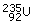?

### Answerhas one proton and no neutrons.has six protons and eight neutrons.has 92 protons and 143 neutrons.

## Question 5

What do the atomic number and mass number of an atom describe?

### Answer

Atomic number describes the number of protons in the nucleus, and hence the type of atom. The mass number describes the total number of protons plus neutrons, together known as nucleons, in the nucleus.

ST174_1

### Take your learning further

Making the decision to study can be a big step, which is why you'll want a trusted University. The Open University has 50 years’ experience delivering flexible learning and 170,000 students are studying with us right now. Take a look at all Open University courses.

If you are new to University-level study, we offer two introductory routes to our qualifications. You could either choose to start with an Access module, or a module which allows you to count your previous learning towards an Open University qualification. Read our guide on Where to take your learning next for more information.

Not ready for formal University study? Then browse over 1000 free courses on OpenLearn and sign up to our newsletter to hear about new free courses as they are released.

Every year, thousands of students decide to study with The Open University. With over 120 qualifications, we’ve got the right course for you.

Request an Open University prospectus371